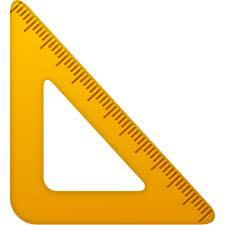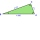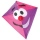# Right Δ

A right triangle has the length of one leg 11 cm and length of the hypotenuse 61 cm. Calculate the height of the triangle.

Result

vc =  10.8 cm

#### Solution:

$a = 11 \ cm \ \\ c = 61 \ cm \ \\ b = \sqrt{ c^2-a^2 } = 60 \ cm \ \\ \ \\ S = S \ \\ \dfrac{1}{2} c v_c = \dfrac{1}{2} a b \ \\ c v_c = a b \ \\ c v_c = a v_c = \dfrac{a}{c} b = \dfrac{ 11}{ 61} \cdot 60 = 10.8 \ \text{cm}$

Try calculation via our triangle calculator.Our examples were largely sent or created by pupils and students themselves. Therefore, we would be pleased if you could send us any errors you found, spelling mistakes, or rephasing the example. Thank you!

Leave us a comment of this math problem and its solution (i.e. if it is still somewhat unclear...):

Showing 1 comment:Math student
Maths

1 year ago  1 LikeTips to related online calculators
Do you have a linear equation or system of equations and looking for its solution? Or do you have quadratic equation?
Pythagorean theorem is the base for the right triangle calculator.
Do you want to convert length units?

## Next similar math problems:

1. The right triangleThe right triangle ABC has a leg a = 36 cm and an area S = 540 cm2. Calculate the length of the leg b and the median t2 to side b.
2. Wall and body diagonalsThe block/cuboid has dimensions a = 4cm, b = 3cm and c = 12cm. Calculates the length of the wall and body diagonals.
3. Concentric circles and chordIn a circle with a diameter d = 10 cm, a chord with a length of 6 cm is constructed. What radius have the concentric circle while touch this chord?
4. Medians in RTThe rectangular triangle ABC has a length of 10 cm and 24 cm. Points P, Q, R are the centers of the sides of this triangle. The perimeter of the PQR triangle is:Calculate the surface of a quadrilateral pyramid, which has a rectangular base with dimensions a = 8 cm, b = 6 cm and height H = 10 cm.
6. Spherical capThe spherical cap has a base radius of 8 cm and a height of 5 cm. Calculate the radius of a sphere of which this spherical cap is cut.
7. Triangular prism - regularThe regular triangular prism is 7 cm high. Its base is an equilateral triangle whose height is 3 cm. Calculate the surface and volume of this prism.
8. Triangular prismCalculate the surface of a regular triangular prism, the edges of the base are 6 cm long and the height of the prism is 15 cm.
9. RT triangle and heightCalculate the remaining sides of the right triangle if we know side b = 4 cm long and height to side c h = 2.4 cm.
10. EmbankmentThe railway embankment 300 m long has a cross section of an isosceles trapezoid with bases of 14 m and 8 m. The trapezoidal arms are 5 m long. Calculate how much m3 of soil is in the embankment?
11. Height of the cuboidCuboid with a rectangular base, measuring 3 cm and 4 cm diagonal has a body 13 centimeters long. What is the height of the cuboid?
12. Cone roofHow many m2 of roofing is needed to cover a cone-shaped roof with a diameter of 10 m and a height of 4 m? Add an extra 4% to the overlays.
13. An equilateralAn equilateral triangle is inscribed in a square of side 1 unit long so that it has one common vertex with the square. What is the area of the inscribed triangle?
14. A kiteChildren have a kite on an 80m long rope, which floats above a place 25m from the place where children stand. How high is the dragon floating above the terrain?
15. FlakesA circle was described on the square, and a semicircle above each side of the square was described. This created 4 "flakes". Which is bigger: the content of the central square or the content of four chips?
16. The conicalThe conical candle has a base diameter of 20 cm and a side of 30 cm. How much dm ^ 3 of wax was needed to make it?
17. KostkaKostka je vepsána do koule o poloměru r = 6 cm. Kolik procent tvoří objem kostky z objemu koule?